20 Oct 2023

Posted on:

03 Oct 2023

0

# Resolved:Where did the instructor get the variance formula from at 2:07?

Where did the instructor get the variance formula from at 2:07?

Instructor
Posted on:

04 Oct 2023

1

Hey Ryan,

Thanks for reaching out!

In the lecture titled "An Overview of Distributions", the lecturer explains that the variance is a measure of how spread out the data is, and introduces the formula for variance. In the formula below, I'll use a discrete random variable X and will assume probabilities of p0 and p1 for the outcomes x0 and x1, respectively :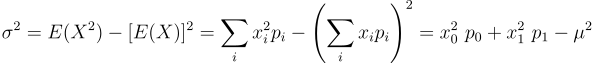We can show that the formula used in the "Bernoulli Distribution" lecture can be rewritten in the form shown above. Let's do that.where we've used that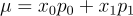and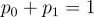Therefore, the formula used in the lecture "An Overview of Distributions" is equivalent to the one used in the "Bernoulli Distribution" lecture.

Hope this helps!

Kind regards,

365 Hristina

Instructor
Posted on:

20 Oct 2023

1

Hey Sayed,

Thank you for reaching out and for your observation!

Note the following derivationIn the derivation above, I've used the following relations: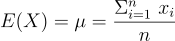From the formula above follows the second substitution made in the derivation, namely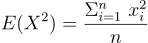Hope this helps!

Kind regards,

365 Hristina